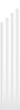Phone: (845) 774-0831    Fax: (845) 774-0835    Email: info@orutilfcu.orgAuto Calculators
How much will my monthly payments be? Calculate How much can I afford for a vehicle? Calculate Which is the best vehicle loan? Calculate Should I take the rebate or special financing? Calculate Should I pay cash or finance? Calculate
Loan Calculators
How can I pay off my debt? Calculate How much will my payments be? Calculate What if I made bi-weekly payments? Calculate
Savings Calculators
What will my savings be worth? Calculate How long will it take for my savings goals to be met? Calculate What will it take to save for a college education? Calculate What will it take to become a millionaire? Calculate What is the cost of waiting to save? Calculate How much more can I save by spending less? Calculate How will different interest rates affect savings? Calculate
Mortgage Calculators
What will my monthly mortgage payment be? Calculate Should I refinance? Calculate
Debt Calculators
What is my debt ratio? Calculate Should I consolidate my debt? Calculate
Retirement Calculators
Am I saving enough for retirement? Calculate How does an IRA, Roth IRA, and Traditional Savings compare? Calculate